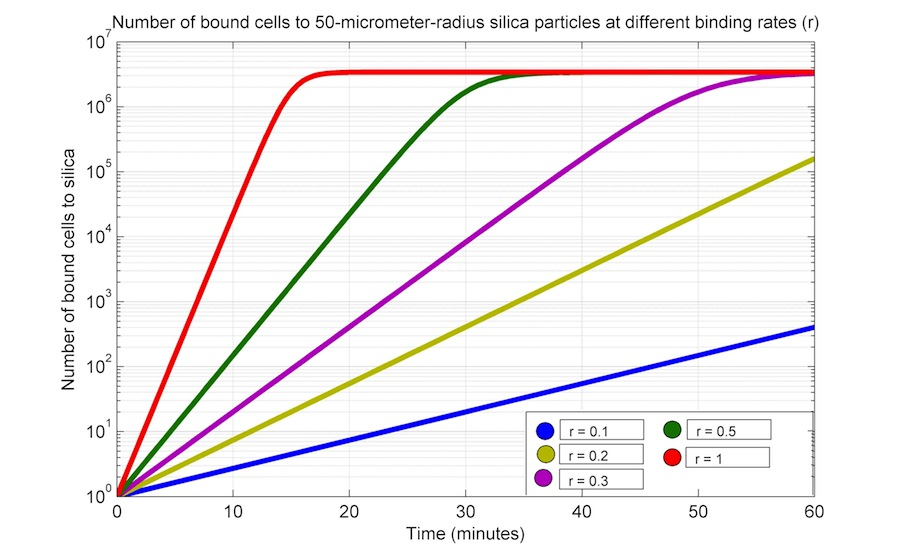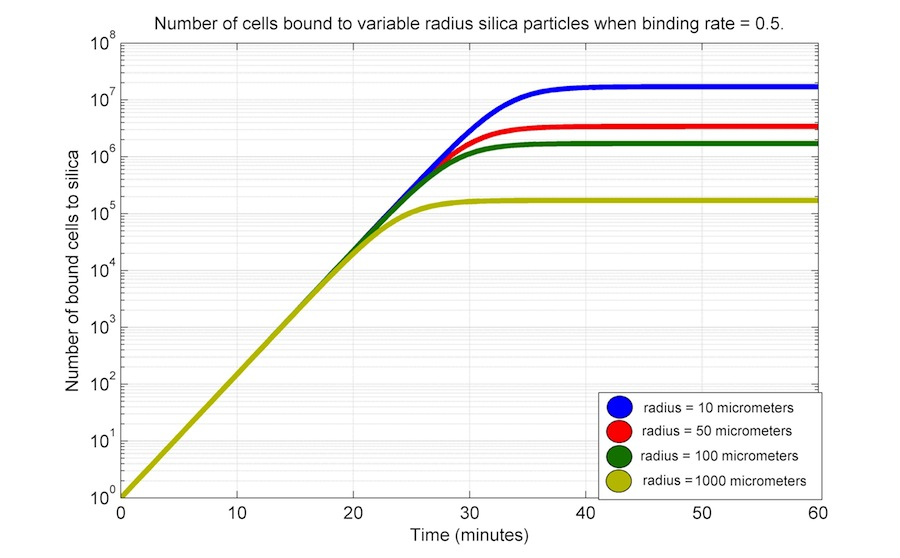# Team:UANL Mty-Mexico/Modeling/Silica binding

iGEM UANL 2012

## Silica binding

The silica binding module of our project is based on the expression of a chimeric transmembranal protein, OmpA-L2. This chimeric protein is composed by an OmpA domain (the transmembranal domain) and a L2 domain (the silica binding domain).

For the mathematical representation of the binding to silica particles, we work at the population level and take into account a Verhulst logistic function to describe the kinetics of this process.

The central assumptions for this model and its limitations are the following: we assume that bacteria are in the stationary phase and that the first binding of a bacterial cell to a silica particle occurs in a faster timescale, so that we can safely assume that it occurs immediately. Finally, this model is restricted to the occasions when the size of the particles is greater than that of the cells.

However, as we do not have the proper experimental data, this model will remain only enunciated, with simulations ran with arbitrary parameters.

## Core model

We start assuming that the silica particles are spheres, so we have that their volume, density, mass and surface area are related in the following way:

\begin{equation} \large V_{particle} = \frac {4 \cdot \pi \cdot radius^{3}}{3} \end{equation} \begin{equation} \large S_{particle} = 4 \cdot \pi \cdot radius^{3} \end{equation} \begin{equation} \large V_{total} = \frac{m}{D_{silica}} \end{equation} \begin{equation} \large N_{particle} = \frac{V_{total}}{V_{particle}} = \frac{3 \cdot m}{4 \cdot \pi D_{silica} \cdot radius^{3}} \end{equation} \begin{equation} \large S_{total} = S_{particle} \cdot N_{particle} = \frac{3 \cdot m}{D_{silica} \cdot radius} \end{equation}

In all these equations, m is the mass (determined experimentally) of our silica particle sample; radius is the radius of a single particle (determined when particles are made); Dsilica is the density of silica; Vparticle and Vtotal are the volume of a single particle and the total volume of the particles (which is can be measured experimentally), respectively; finally, Sparticle and Stotal are the surface area for a single particle and for the total particles considered, respectively.

Now, let us introduce the concept of "Bacterial shadow" (W), which is the amount of the total surface area of the silica particles covered by a single bacterial cell.

In order to determine W, we first assume that the most stable interaction between a cell and a particle is that when the cell lies with the maximum number of contact area (the amount of a single cell surface that is in actual contact with a particle); we'll call this conformation the "whole binding", or WB conformation. All other conformations will be unstable and the system will tend to stabilize when all bound cells are in this whole binding conformation.

We cannot directly assume that all conformations other than the WB will immediately be substituted by a WB, whether by unbinding from the silica particle or by conformation change, mainly because some of them will be very close to the WB conformation and will have similar strength. In fact, we can build a probability distribution that assigns a probability to the bacterial shadow associated with every single possible conformation. In this way, at a given moment there will be cells bound in different ways, most of them in those conformations that are close to the WB conformation.

But as this probabilistic analysis is beyond the scope of the model presented for the iGEM Latin America Regional Jamboree, we will assume that the binding strength is practically the same for the WB conformation and those that closely resemble it, so that the later will fall in the WB conformation in a faster time scale that allows us to assume that this change is immediate.

On the other hand, we assume as well that all the other, more unstable conformations will be destroyed and replaced also in a faster time scale.

In this way, at any moment, the bacterial shadow cast by a single cell can be thought to be the one that is cast by a WB conformation bacteria. This shadow will be an ellipse with length and width equal to that of a single cell and can be calculated with the ellipse area formula as follows:

\begin{equation} \large W = \frac{\pi \cdot width \cdot length}{4} \end{equation}

The total spaces available for cells to bind (K) to our silica particles will be the division of the total silica particle surface by the bacterial shadow:

\begin{equation} \large K = \frac{S_{total}}{W} = \frac{12 \cdot m}{\pi \cdot D_{density} \cdot radius \cdot length \cdot width} \end{equation}

Finally, as cells do not bind in a regular order that actually occupies all the available spaces, we'll run simulations considering different efficiencies of occupancy.

### ODEs

The kinetics for the binding of cells to the silica surface is represented as a Verhulst logistic function.

\begin{equation} \large \frac{dB_{cells}}{dt} = r \cdot B_{cells} (1 - \frac{B_{cells}}{K}) \end{equation}

Where Bcells are the bound cells; r is the binding rate; finally, K is the total spaces available in the total silica surface (as calculated in equation 7).

As we do not have experimental data, we performed a series of time simulations varying the value of r and K; the later was varied by modifying the particle radius and holding constant the following parameters: bacterial length and width, and particle mass (1000 μg); D_silica is 2.65 × 10-6 μg μm-3. All units are in μg, μm3 and min.The last simulation shows that the particle radius has an inverse relationship with the number of bound cells; this can be explained by the fact that the particle surface area decreases because the total mass is held constant.